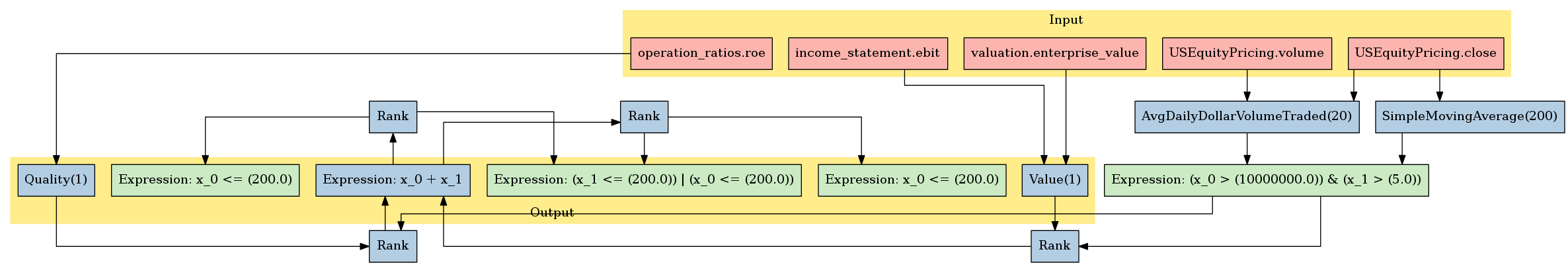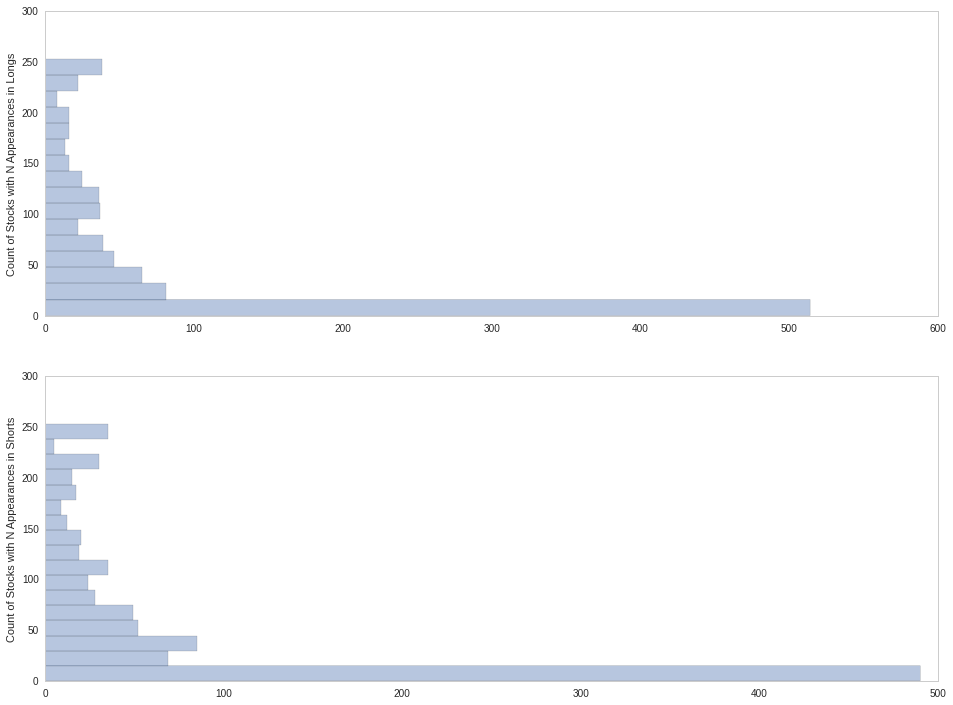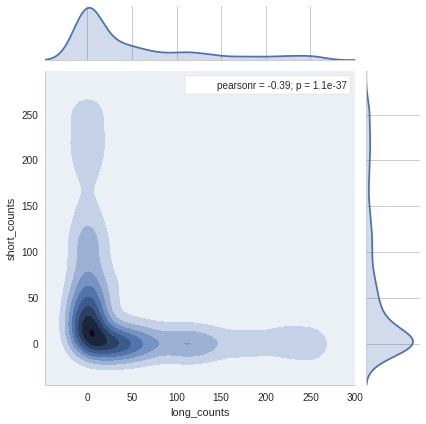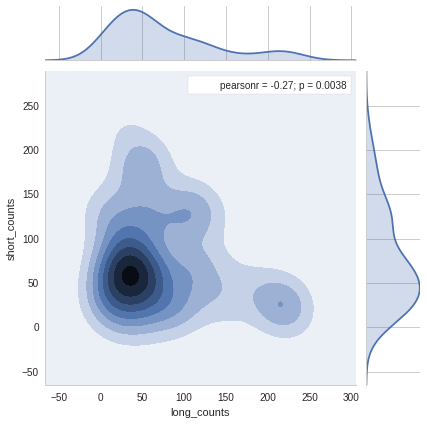Notebook

# Analyzing a Long/Short Equity Pipeline¶

One of the primary benefits of the Pipeline API is that Filter, Factor, and Pipeline definitions are transferrable between backtesting and research. This makes it easy to develop and analyze a Pipeline with an interactive workflow, moving the final product to the backtester only when we're ready to incorporate our work into a full trading strategy.

In this notebook, we show how to run and analyze a pipeline describing a simple long/short portfolio.

We build a Pipeline that ranks assets based on combined Value/Quality metrics, constructing a long portfolio out of the top 200 assets and a short portfolio out of the bottom 200 assets. Ranks are performed after performing an initial screen that removes assets that fail to meet basic liquidity and stability criteria.

We then use pandas and seaborn to analyze the results of our Pipeline. In our analysis we show how to do the following:

• Verify that our target portfolio contains exactly 200 longs and 200 shorts every day.
• Visualize the distribution of appearance counts in longs and shorts.
• Visualize the top 20 stocks that appear in our longs and shorts.

#### Everything in this cell can be transferred to the Quantopian backtest environment.¶

In :
import numpy as np
from quantopian.pipeline import Pipeline
from quantopian.pipeline.data import morningstar
from quantopian.pipeline.data.builtin import USEquityPricing
from quantopian.pipeline.factors import CustomFactor, SimpleMovingAverage

class Value(CustomFactor):
inputs = [morningstar.income_statement.ebit,
morningstar.valuation.enterprise_value]
window_length = 1

def compute(self, today, assets, out, ebit, ev):
out[:] = ebit[-1] / ev[-1]

class Quality(CustomFactor):

# Pre-declare inputs and window_length
inputs = [morningstar.operation_ratios.roe,]
window_length = 1

def compute(self, today, assets, out, roe):
out[:] = roe[-1]

inputs = [USEquityPricing.close, USEquityPricing.volume]

def compute(self, today, assets, out, close_price, volume):
out[:] = np.mean(close_price * volume, axis=0)

def make_pipeline():
"""
Create and return our pipeline.

We break this piece of logic out into its own function to make it easier to
test and modify in isolation.

In particular, this function can be copy/pasted into research and run by itself.
"""
pipe = Pipeline()

# Basic value and quality metrics.
value = Value()
quality = Quality()

# We only want to trade relatively liquid stocks.
# Build a filter that only passes stocks that have $10,000,000 average # daily dollar volume over the last 20 days. dollar_volume = AvgDailyDollarVolumeTraded(window_length=20) is_liquid = (dollar_volume > 1e7) # We also don't want to trade penny stocks, which we define as any stock with an # average price of less than$5.00 over the last 200 days.
sma_200 = SimpleMovingAverage(inputs=[USEquityPricing.close], window_length=200)
not_a_penny_stock = (sma_200 > 5)

# Before we do any other ranking, we want to throw away these assets.
initial_screen = (is_liquid & not_a_penny_stock)

# Construct and add a Factor representing the average rank of each asset by our
# value and quality metrics.
# By applying a mask to the rank computations, we remove any stocks that failed
# to meet our initial criteria **before** computing ranks.  This means that the
# stock with rank 10.0 is the 10th-lowest stock that passed initial_screen.
combined_rank = (
)

# Build Filters representing the top and bottom 200 stocks by our combined ranking system.
# We'll use these as our tradeable universe ech
longs = combined_rank.top(200)
shorts = combined_rank.bottom(200)

# The final output of our pipeline should only include
# the top/bottom 200 stocks by our criteria.
pipe.set_screen(longs | shorts)

return pipe

In :
pipe = make_pipeline()


#### The interactivity of the research environment allows us to visualize our computations in new ways.¶

In :
pipe.show_graph('png')

Out:#### In the backtester, we would call attach_pipeline(pipe) in our initialize function.¶

Under the hood, the backtester calls run_pipeline on dynamically-sized chunks of dates, making (hopefully intelligent) tradeoffs between memory usage and execution time, and ensuring that algorithms aren't exposed to lookahead bias by gaining early access to pre-fetched data. See Pipeline in Research: What are the runtime limits? for an in-depth look at how this works.

In research we provide raw access to the run_pipeline function, which accepts a Pipeline object, a start_date, and an end_date.

In :
from quantopian.research import run_pipeline

In :
# This takes a few minutes.
results = run_pipeline(pipe, '2011', '2012')
results

Out:
combined_rank longs quality shorts value
2011-01-03 00:00:00+00:00 Equity(122 [ADI]) 2113 True 0.072181 False 0.034893
Equity(168 [AET]) 1988 True 0.048921 False 0.041079
Equity(185 [AFL]) 2128 True 0.065208 False 0.039891
Equity(205 [AGN]) 55 False -0.140456 True -0.036802
Equity(216 [HES]) 2245 True 0.076690 False 0.052778
Equity(300 [ALK]) 2398 True 0.121585 False 0.097667
Equity(328 [ALTR]) 2081 True 0.123602 False 0.026429
Equity(337 [AMAT]) 2167 True 0.063182 False 0.050222
Equity(368 [AMGN]) 1968 True 0.052327 False 0.034416
Equity(374 [AMLN]) 79 False -0.136389 True -0.022064
Equity(559 [ASH]) 122 False -0.030835 True -0.030089
Equity(595 [GAS]) 2223 True 0.074156 False 0.049713
Equity(600 [OA]) 2233 True 0.104823 False 0.036463
Equity(607 [ATML]) 1926 True 0.297228 False 0.020198
Equity(612 [ATO]) 446 False 0.000684 True 0.008046
Equity(693 [AZO]) 2010 True 0.906995 False 0.021554
Equity(698 [BA]) 2172 True 0.222281 False 0.027908
Equity(869 [BID]) 2280 True 0.136103 False 0.036669
Equity(915 [BKE]) 2150 True 0.085545 False 0.033687
Equity(939 [BLL]) 2148 True 0.149151 False 0.028285
Equity(980 [BMY]) 2084 True 0.059991 False 0.039744
Equity(1103 [BRY]) 327 False -0.002906 True 0.003966
Equity(1332 [CCE]) 2121 True 0.182904 False 0.026251
Equity(1343 [CCK]) 2327 True 1.032787 False 0.033899
Equity(1374 [CDE]) 180 False -0.013810 True -0.012493
Equity(1376 [CAH]) 2039 True 0.056072 False 0.037674
Equity(1402 [CEF]) 2432 True 0.208714 False 0.066276
Equity(1416 [CEPH]) 2094 True 0.055788 False 0.047386
Equity(1539 [CI]) 2168 True 0.062417 False 0.052621
Equity(1551 [CINF]) 435 False 0.005624 True 0.005919
... ... ... ... ... ... ...
2012-01-03 00:00:00+00:00 Equity(35359 [DAN]) 2354 True 0.098598 False 0.065095
Equity(35531 [CPN]) 234 False -0.005182 True -0.006690
Equity(35763 [MAKO]) 104 False -0.102289 True -0.009924
Equity(35902 [PM]) 2072 True 0.819797 False 0.024280
Equity(35961 [NOG]) 265 False -0.005242 True -0.001665
Equity(36346 [LO]) 2195 True 9.280000 False 0.028434
Equity(36448 [LPS]) 2214 True 0.083408 False 0.040285
Equity(36628 [GTAT]) 2418 True 0.130012 False 0.082029
Equity(36763 [WPRT]) 166 False -0.070863 True -0.001583
Equity(39073 [CIE]) 197 False -0.017196 True -0.005976
Equity(39095 [CHTR]) 375 False -0.095280 True 0.011571
Equity(39347 [ST]) 214 False -0.034753 True 0.000585
Equity(39499 [VIP]) 2124 True 0.055162 False 0.059327
Equity(39546 [LYB]) 2190 True 0.061257 False 0.065922
Equity(39626 [EXPR]) 2398 True 0.371627 False 0.046642
Equity(39797 [OAS]) 342 False 0.002891 True 0.001906
Equity(39840 [TSLA]) 65 False -0.240569 True -0.017217
Equity(39905 [KKR]) 2451 True 0.148400 False 1.187382
Equity(39921 [QLIK]) 226 False -0.016688 True -0.001930
Equity(39960 [MCP]) 186 False -0.021375 True -0.005539
Equity(39994 [NXPI]) 147 False -0.114286 True -0.000722
Equity(40376 [TFM]) 201 False -0.032224 True -0.001026
Equity(40606 [SWFT]) 324 False 0.009801 True -0.010720
Equity(40616 [MMI]) 265 False -0.006496 True -0.000121
Equity(40755 [NLSN]) 396 False 0.001039 True 0.006541
Equity(41462 [MOS]) 2070 True 0.057388 False 0.043186
Equity(41601 [RATE]) 348 False -0.007616 True 0.006133
Equity(41770 [CJES]) 2066 True 0.132503 False 0.026460
Equity(42021 [XLS]) 2096 True 0.049425 False 0.101889
Equity(42118 [GRPN]) 37 False -46.892494 True -0.027629

101200 rows × 5 columns

### Verify that we have 400 assets in our universe each day.¶

In :
# Verify that we get 400 assets in our universe each day.
assets_per_day = results.groupby(level=0).size()
assets_per_day.describe()

Out:
count    253
mean     400
std        0
min      400
25%      400
50%      400
75%      400
max      400
dtype: float64

### Verify that we have 200 longs and 200 shorts each day.¶

In :
long_short_count_by_day = results[['longs', 'shorts']].groupby(level=0).sum()
long_short_count_by_day.describe()

Out:
longs shorts
count 253 253
mean 200 200
std 0 0
min 200 200
25% 200 200
50% 200 200
75% 200 200
max 200 200

### Plot the distribution of assets with N appearances in Longs/Shorts.¶

In :
import seaborn as sns
import matplotlib.pyplot as plt

# Create a 2 x 1 vertical grid of plotting areas.
fig, axes = plt.subplots(2, 1, figsize=(16, 12))

# Compute counts of long/short appearances, grouped by asset.
long_counts_by_asset = results['longs'].groupby(level=1).sum().order()
short_counts_by_asset = results['shorts'].groupby(level=1).sum().order()

# Plot long counts on first axis.
sns.distplot(long_counts_by_asset, ax=axes, kde=False, vertical=True,
axlabel="Count of Stocks with N Appearances in Longs")

# Plot short counts on second axis.
sns.distplot(short_counts_by_asset, ax=axes, kde=False, vertical=True,
axlabel="Count of Stocks with N Appearances in Shorts")

# Remove grid lines from axes.  They look bad with the horizontal bar chart.
axes.grid(False)
axes.grid(False)In :
long_every_day = long_counts_by_asset[long_counts_by_asset == 253]
for asset in long_every_day.index:
print asset

Equity(22275 [SYT])
Equity(4487 [LLY])
Equity(12652 [DLTR])
Equity(32603 [WU])
Equity(13197 [FCX])
Equity(5061 [MSFT])
Equity(869 [BID])
Equity(4246 [KLAC])
Equity(13017 [DISH])
Equity(25555 [ACN])
Equity(36628 [GTAT])
Equity(21475 [BAM])
Equity(25317 [DELL])
Equity(3014 [FRX])
Equity(22139 [MCO])
Equity(1595 [CLF])
Equity(36346 [LO])
Equity(337 [AMAT])
Equity(25948 [TRW])
Equity(2407 [EV])
Equity(19954 [AZN])
Equity(35902 [PM])
Equity(3951 [INTC])
Equity(3212 [GILD])
Equity(12691 [LMT])
Equity(20177 [BPO])
Equity(24831 [ESI])
Equity(8050 [VSH])

In :
short_every_day = short_counts_by_asset[short_counts_by_asset == 253]
for asset in short_every_day.index:
print asset

Equity(374 [AMLN])
Equity(19249 [RRC])
Equity(7844 [USG])
Equity(10509 [SHAW])
Equity(10409 [HGSI])
Equity(17767 [TLM])
Equity(19497 [MMR])
Equity(15005 [PSS])
Equity(27411 [LEAP])
Equity(35531 [CPN])
Equity(21612 [DNDN_Q])
Equity(35961 [NOG])
Equity(5969 [PHM])
Equity(25781 [NG])
Equity(34873 [PCX])
Equity(6413 [REGN])
Equity(39960 [MCP])
Equity(2500 [ELN])
Equity(4531 [LPX])
Equity(16453 [CIEN])
Equity(6612 [RYL])
Equity(14986 [ONXX])
Equity(1663 [CRK])
Equity(20281 [SBAC])
Equity(1374 [CDE])
Equity(11598 [AIV])
Equity(4831 [MGM])


### Assets that we longed and shorted at least once each.¶

In :
import pandas as pd

combined_counts = pd.DataFrame(
data={
'long_counts': long_counts_by_asset,
'short_counts': short_counts_by_asset
},
)


Out:
long_counts short_counts
Equity(2 [AA]) 0 2
Equity(24 [AAPL]) 241 0
Equity(62 [ABT]) 110 0
Equity(88 [ACI]) 0 64
In :
sns.jointplot(combined_counts.long_counts, combined_counts.short_counts, kind='kde')

Out:
<seaborn.axisgrid.JointGrid at 0x7f409983afd0>In :
mixed_assets = combined_counts[(combined_counts.long_counts > 0) & (combined_counts.short_counts > 0)]
mixed_assets

Out:
long_counts short_counts
Equity(239 [AIG]) 223 9
Equity(559 [ASH]) 65 49
Equity(755 [BC]) 127 78
Equity(845 [BGG]) 6 32
Equity(1103 [BRY]) 104 149
Equity(1402 [CEF]) 98 11
Equity(1581 [CKH]) 30 88
Equity(2010 [CVH]) 64 30
Equity(2568 [EP]) 30 33
Equity(2602 [EA]) 77 138
Equity(2893 [FMC]) 49 54
Equity(3103 [GAS]) 30 12
Equity(3131 [GCO]) 13 58
Equity(3384 [GT]) 30 223
Equity(3660 [HRB]) 120 133
Equity(3990 [IPG]) 127 126
Equity(4118 [JCP]) 41 36
Equity(4580 [LUK]) 223 30
Equity(4684 [MBI]) 223 30
Equity(4794 [MENT]) 50 31
Equity(5121 [MU]) 21 53
Equity(5520 [NWL]) 57 74
Equity(5626 [OI]) 34 113
Equity(5643 [OLN]) 59 51
Equity(5907 [PDCE]) 1 137
Equity(6624 [SAFM]) 238 6
Equity(6736 [SMG]) 136 117
Equity(6930 [HSH]) 142 40
Equity(7203 [MW]) 63 57
Equity(7233 [SVU]) 130 72
... ... ...
Equity(24789 [PLCE]) 168 64
Equity(25305 [AXS]) 51 67
Equity(26169 [SHLD]) 201 52
Equity(26265 [GHL]) 33 92
Equity(26440 [WCG]) 41 28
Equity(27409 [DSW]) 27 68
Equity(27653 [LCC]) 223 30
Equity(27822 [UA]) 20 35
Equity(27886 [BAS]) 49 74
Equity(27997 [WNR]) 104 61
Equity(28051 [UAL]) 114 139
Equity(28078 [CROX]) 86 30
Equity(28083 [XCO]) 27 223
Equity(32367 [AWH]) 12 82
Equity(32660 [SFLY]) 50 180
Equity(32856 [CSIQ]) 75 23
Equity(32887 [HTZ]) 41 68
Equity(33729 [DAL]) 30 174
Equity(33856 [CLR]) 104 119
Equity(33955 [LDK]) 156 40
Equity(33959 [JAZZ]) 99 21
Equity(34114 [SPRD]) 223 17
Equity(34334 [VR]) 30 57
Equity(34440 [CXO]) 105 75
Equity(34930 [LFT]) 11 30
Equity(35006 [SD]) 104 119
Equity(35140 [TC]) 101 122
Equity(35359 [DAN]) 45 30
Equity(35651 [SOL]) 140 30
Equity(38915 [LSTZA]) 156 9

117 rows × 2 columns

#### Plot the distribution of appearance counts for assets with at least one long and one short appearance.¶

In :
sns.jointplot(mixed_assets.long_counts, mixed_assets.short_counts, kind='kde')

Out:
<seaborn.axisgrid.JointGrid at 0x7f40989e88d0>In [ ]: